A large number of laboratories use absorbance when analyzing in experimental manipulations. By measuring how much light passes through, you can measure how much of a substance is present in the solution. Alternatively, it can be used to check how far a reaction has progressed.

When experimenting with biological systems, we frequently use absorbance to analyze them. In essence, we can measure how much light penetrates, which allows us to see how compounds and enzymes work, and to measure the concentration of substances.

By checking how much light penetrates the solution, we can determine if the concentration is high or low. Naturally, if the color is darker, we can assume that there are more substances present.

Terms such as the UV-visible absorption spectrum and Beer–Lambert law, you may think the concept and principle of absorbance are very difficult. So, we can understand it as a method to measure the amount of a substance in a solution by making use of the property that light is less likely to pass through when the concentration is high.

## Conjugation in the Structural Formula Absorbs Light

There are so many colored liquids around us. Why do they have these colors? It’s because it contains some compounds.

If it doesn’t contain any compounds, it’s pure water, therefore transparent. On the other hand, the liquid is colored as a result of the presence of some organic compound in it. It is important to understand that a colored liquid always contains some kind of compound.

Colored liquids always contain compounds, such as heavy metals or organic compounds with conjugated structures. Note that most colored liquids contain compounds with conjugated structures. For example, a compound that absorbs ultraviolet or visible light has the following structural formula.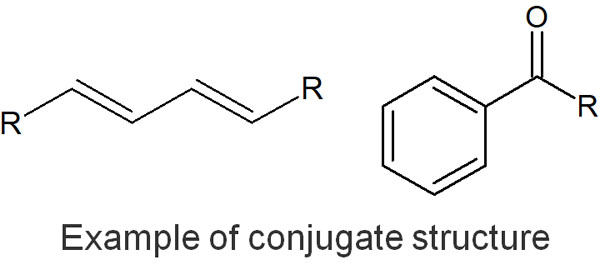For example, sunscreen creams contain substances that absorb UV rays. In tea, there are many organic compounds called polyphenols. As a result of the presence of these compounds, the product absorbs UV rays and the solution has a color.

The most famous conjugated structure is the benzene ring. Any compound with a benzene ring will have a UV-visible absorption spectrum. In the case of the benzene ring, it absorbs UV light, which is why the peak of the UV-visible absorption spectrum appears.

### Measuring Differences in Light Transmission (Absorbance)

The presence of coloration means that the organic compound absorbs the color. Because the compound absorbs light, we perceive the liquid as having a color.

If an organic compound absorbs ultraviolet or visible light, the intensity of the light will be weakened by that amount. Also, if you shine a light on a dark-colored solution, the light that comes out will be weaker by that amount.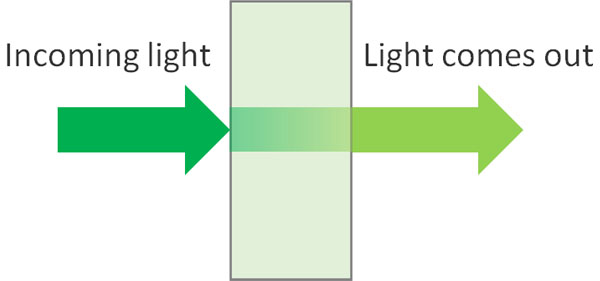It’s easy to imagine that the higher the concentration of compounds in the solution, the more light it absorbs and the less intense the light that comes out.

For example, if you use a blackout sheet, it will absorb all the light. However, if the black color is lighter, the light will pass through and reach you. On the other hand, the darker the black color, the more light will be blocked. In the same way, think of the UV-visible absorption spectrum as focusing on the transmission of light.

### The Principle Behind the UV-Visible Absorption Spectrum Is the Emission of Energy

Why do organic compounds absorb ultraviolet (UV) and visible light (VIS)? Different substances absorb different wavelengths of light. The UV-VIS absorption spectrum describes the wavelengths that a solution absorbs.

Which light is absorbed and appears as the UV-VIS absorption spectrum depends on the compound dissolved in the solution. But why does it appear in the UV-visible absorption spectrum?

It is due to the excited state of the electron.

Atoms have electrons, and electrons are in a stable state (ground state). When energy is added from outside, the electron temporarily enters a high-energy state. This is the excited state.

The transfer of electrons to higher energy orbitals instead of their original orbitals is called an electronic transition. When light energy hits a compound, it causes an electronic transition. However, molecules dislike high-energy states, so they try to return to the original stable state after an electronic transition. When the molecule returns to the stable state (ground state), it emits light and heat as energy.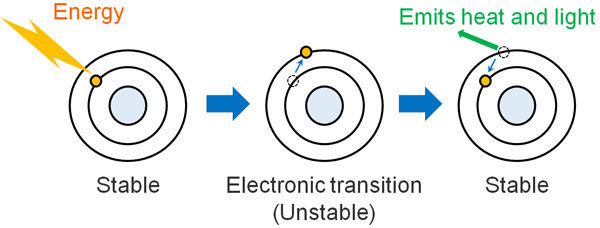When light hits a solution, there is a reason why the light is weakened after transmission. It’s because the compound absorbs the light energy and then releases the energy as heat or light. Naturally, the darker the solution, the more light is absorbed, so the light after transmission becomes weaker.

As for blackout sheets, the sheets are hot after blocking a lot of light. This is because it absorbs light energy and stores a lot of energy as heat.

## Absorbance Is Related to Concentration and Distance

This property is applied in scientific experiments. By measuring how much light is absorbed as the light passes through a liquid, we can measure the amount of substance contained or the progress of the reaction.

There are two factors involved in absorbance: concentration and distance. The denser the concentration, the more light will be absorbed. In addition, the longer the distance, the more light is absorbed, even if the solution has the same concentration.

For example, suppose there is a solution in which the light intensity drops to 50% after the light passes through. If the length of this solution is doubled, the light will be 25% after transmission.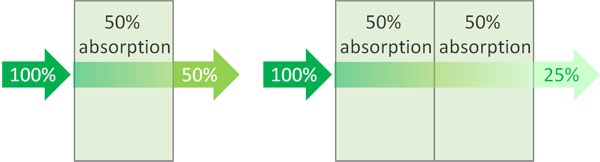First 50% of the light is absorbed, then 50% of the light is absorbed. The result is the following.

• 0.5 × 0.5 = 0.25 (25%)

Understand that as the distance increases, the intensity of the light weakens as an exponential function.

### Understanding Beer–Lambert Law

Once you learn these details, it is easy to understand Beer–Lambert law. Many people try to explain Beer–Lambert law by using difficult symbols and formulas. However, these do not make sense.

In Beer–Lambert law, the two names are combined.

It was discovered that when the concentration is the same, the absorbance is proportional to the distance (the length of light transmitted). This is called Lambert law (Bouguer law).

On the other hand, when the distance (length of light transmission) is the same, the absorbance depends on the concentration (molar concentration of the solute) of the solution. This is called Beer law.

Beer–Lambert law is a combination of Lambert law (Bouguer law) and Beer law. However, it only states the obvious: the denser the solution is and the longer the distance, the weaker the light after transmission.

### Use Absorbance, Not Transmittance

What is the reason for using absorbance (A) instead of transmittance? This is because the graph does not form a straight line when using transmittance.

By measuring the intensity of the output light (I0) and the intensity of the transmitted light (I), the transmittance can be easily measured. The transmittance is shown below.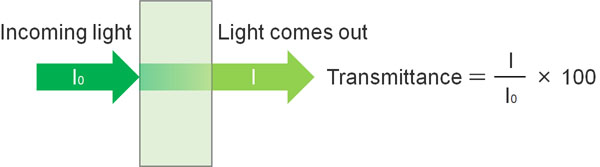The graph will bend when you use transmittance to give a number. We explained earlier that as the distance increases, the light gets weaker, like an exponential function: if you have 50% transmission, then when you double the distance, you don’t get zero transmission; you get 25% transmission.

So instead of transmittance, we use logarithm. If you add a minus sign to the logarithm, you will get a straight line graph.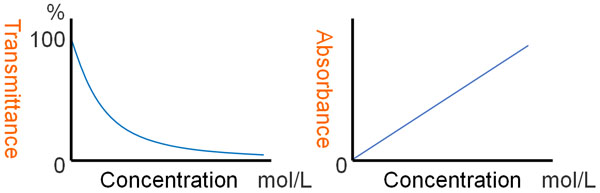Absorbance is the logarithm of the transmittance, plus a negative number. Because of the use of absorbance, the line in the graph is straightened, and the experimental data can be read.

Beer–Lambert law also uses other equations. The formula is as follows.

• A (Absorbance) = εcl
• ε (Epsilon): Molar absorption coefficient
• c: Concentration of the solution
• l: Distance of light

In Beer–Lambert law, we explained that absorbance is proportional to concentration and distance. Therefore, the absorbance can be calculated by multiplying the concentration of the solution (c) and the distance of the light (l). Although the formula may seem difficult, it is a very simple one.

ε (epsilon) is called the molar absorbance coefficient. When c is 1 mol/L, and l is 1 cm, the molar absorbance is ε.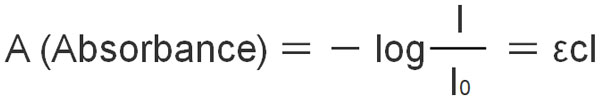If you are shown this formula at first, you will not understand what it means. However, once you understand Beer–Lambert and the meaning of absorbance, you will be able to understand it easily.

## Determine the Wavelength of Measurement Using the UV-Visible Absorption Spectrum

These are the principles of Beer–Lambert law of absorbance measurement. So, how can we analyze it?

First of all, we have to know what kind of light the target compound absorbs. So let’s research the UV-visible absorption spectrum. By exposing the compound to ultraviolet and visible light by a machine, the UV-visible absorption spectrum will appear. It looks something like the following.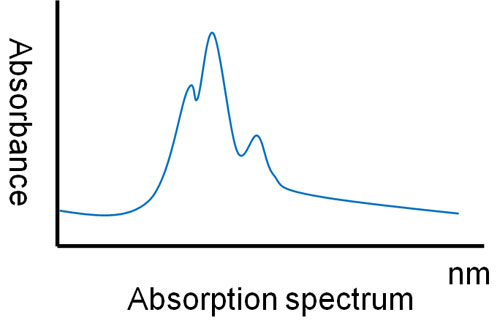From the data in the UV-visible absorption spectrum, decide which wavelengths of light you want to use in your experiment.

Usually, We use the wavelength that has the highest absorbance. This is the point at which light is easily absorbed, and thus data can be obtained from the experiment.

### Measuring the Absorbance Will Give You the Concentration of the Solution

After determining the wavelengths to be used by the UV-visible absorption spectrum, what is the next step in the experiment?

The experiment varies from person to person. Some people want to know the concentration of a substance, while others want to know the progress of a reaction. But in any case, we can calculate the concentration of the solution by measuring the absorbance. We then produce the data.

In laboratories involved in biochemistry, including cell experiments, absorbance measurements are frequently used. For example, the following small plates are used to examine the degree of coloration.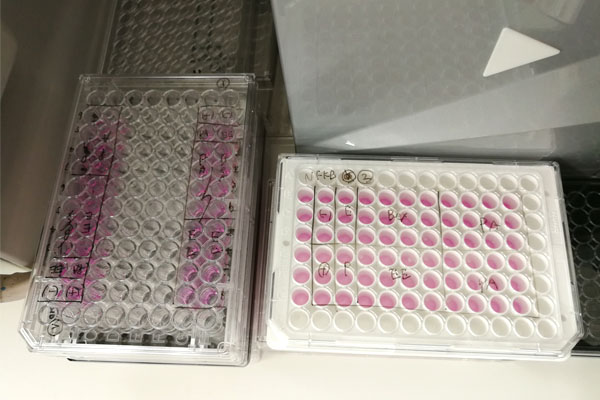Depending on how much color is generated, the concentration of a substance in the solution can be determined. Since we only measuring absorbance, the data that comes out is a list of numbers (absorbance). So by organizing the absorbance numbers, you will be able to get your data.

A great number of scientific experiments are being conducted in laboratories that make use of color differences, such as the quantification of substances, enzyme reactions, and the use of cells. By using the absorption of ultraviolet light and the use of chromophores, we can measure how much of a compound is present there by the degree of light absorption. As a result, we are able to collect experimental data.

For example, there are reagents that react with glucose (sugar) to produce color. If blood is collected and colored by reacting with the reagent, it is possible to measure the blood glucose level according to the intensity of the color. The measurement of absorbance is applied in medicine.

It is also used in scientific experiments and measurements in many other situations using Beer–Lambert law. Although it only measures absorbance as the transmission of light, it is one of the measurement technologies used in all aspects of our lives.

### Proteins, For Example, May Themselves Absorb Light

In addition, substances themselves often absorb light when they are measured. There are few compounds that have a dye, but there are many compounds that absorb UV light. As mentioned above, if they have a conjugated structure, they will absorb UV light.

For example, in proteins, the UV-visible absorption spectrum can be seen. Proteins have peptide bonds, which absorb UV light.

There are also aromatic amino acids (tyrosine and tryptophan) in proteins. Because they have a benzene ring in their structural formula, they have a conjugated structure and absorb UV light. For this reason, the concentration of proteins can be estimated by measuring the absorbance.

-In General, Oher Than Ultraviolet Light Are Used to Measure Absorbances

Many organic substances, including proteins, have a conjugated structure and absorb UV light. Therefore, it is common to use visible light as the light source for absorbance analysis.

Although UV light can be used as a light source, any organic compound with a conjugated structure absorbs UV light. Therefore, when multiple substances are mixed in a solution, not only your target compound but also other substances will absorb UV light, making it difficult to obtain accurate data.

So we use other light (visible colors). For example, let’s assume it reacts with a reagent to produce a red color. Shine the light on the solution. Then we can measure only the compounds that are dyed red by the reagent. Depending on the intensity of the red color, the concentration of the compound can be measured.

By using reagents that are visibly colored, UV-visible spectroscopy is useful in many biochemical experiments.

## Understanding the Principles of Absorption Spectra and Absorbance by UV-Visible Spectroscopy

By using UV-visible spectroscopy and using the UV-visible absorption spectrum, we can find out what wavelength of light the compound absorbs. We can then use Beer–Lambert law and measure the absorbance to get the desired number.

It is not possible to understand the principle and meaning of Beer–Lambert law by looking at the formula alone. There is no point in using a difficult mathematical formula. The concept of absorbance can be understood simply by knowing multiplication and logarithm.

These absorbance measurements are not only used in the laboratory, but are also used in all kinds of research facilities. This includes government agencies, hospitals, and so many other institutions. It is a simple and common method to determine the degree of absorption of light and give the concentration of a target compound.

The measurement of absorbance is used in a great many situations. By measuring absorbance, you should be able to obtain the desired data.